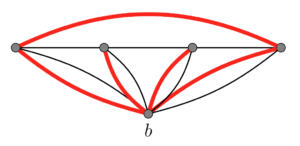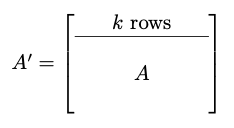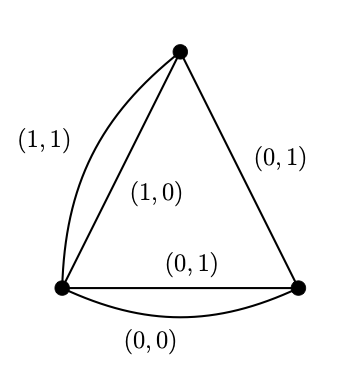# Online Talk: Nathan Bowler

Time: Wednesday, Sep 20 at 3pm ET
Zoom: https://gatech.zoom.us/j/8802082683

Speaker: Nathan Bowler, Universität Hamburg
Title: The $K_4$ game

Abstract: Two players alternately claim edges of a complete graph on infinitely many vertices. The first to claim all edges of a $K_4$ wins. Can the first player force a win? I will explain the history of this question, why it is harder than it seems, and how to win this game.

# Using matroids to prove min-max theorems

There is a wonderful matroidal proof of the Tutte-Berge formula for the maximum size of a matching . Today we discuss another min-max theorem  which has a similar matroidal proof. Tantalizingly, the two theorems do not (yet?!) have a common generalization. This theorem was just posted to arXiv by yours truly – it arose from joint work with Jim Geelen and Paul Wollan on vertex-minors, which I’ll have to discuss later!

Matchings and the proof strategy

First we outline the proof in the case of matchings. It’s more convenient to work in the “dual setting”. So, given a graph $G$, we write $\textrm{defect}(G)$ for the minimum number of vertices left uncovered by a matching. So, where $\nu(G)$ denotes the size of a maximum matching of $G$, we have $\textrm{defect}(G) = |V(G)|-2\nu(G)$.

We write $\textrm{odd}(G)$ for the number of components of a graph $G$ with an odd number of vertices. Note that for any $X \subseteq V(G)$, we have $\textrm{defect}(G) \ge \textrm{odd}(G-X)-|X|$, since all but $|X|$ of the odd components must contribute towards the defect. The min-max theorem says that in fact equality can be achieved.

Theorem (Tutte-Berge Formula): For any graph $G$,
$$\textrm{defect}(G) = \max_{X \subseteq V(G)} \left(\textrm{odd}(G-X)-|X|\right).$$

Here is a rough roadmap on using matroids to prove min-max theorems like this one. This strategy is due to Chudnovsky, Geelen, Gerards, Goddyn, Lohman, and Seymour , who use it to prove a theorem which does generalize the Tutte-Berge Formula.

1. Find a matroid whose rank equals the defect. In this case, sets which are of the form $V(G)\setminus V(M)$ for some maximum matching $M$ form the bases of a matroid. This is the dual of the well-known matching matroid.
2. Reduce to the case that the matroid is loopless. In this case, no vertex-minimum counterexample can contain a loop vertex $v$. Otherwise, we would have $\textrm{defect}(G)<\textrm{defect}(G-v)$, and so we could add $v$ to the set $X$ obtained for $G-v$ to get a “good set” for $G$ and thus a contradiction.
3. Use transitivity of parallel pairs to complete the proof. In this case, each component of $G$ has rank $1$ by transitivity of parallel pairs and the fact that for each edge $uv \in E(G)$, the vertices $u$ and $v$ are parallel (that is, there is no maximum matching which leaves both $u$ and $v$ uncovered). So we can take $X = \emptyset$ since each component is odd and contributes $1$ towards the defect.

The new min-max theorem

The new min-max theorem is for “rooted Eulerian signed graphs”, which is a bit of a mouthful. The point is that we have a (multi-)graph $G$ which is Eulerian (connected and with every vertex of even degree), a specified vertex $b \in V(G)$ called the root, and a specified set of edges $S \subseteq E(G)$ called the signature. It is helpful to think of the edges in $S$ as being “odd” and the other edges as being “even”.

Since $G$ is Eulerian, it is possible to partition its edge-set into exactly $\textrm{degree}(b)/2$ many parts, each of which is the edge-set of a trail that begins and ends at $b$. We call such a trail a $b$-trail, and we say that it is odd if it contains an odd number of edges from $S$. We are interested in the following maximization problem.

Problem: What is the maximum number of odd trails in a collection of $\textrm{degree}(b)/2$ many $b$-trails which partitions $E(G)$?

We call this the flooding number and denote it by $\widetilde{\nu}$. The min-max theorem for $\widetilde{\nu}$ is somewhat technical to state, so we refer the reader to . However, here is an example of our version of an “odd component”. The thick red edges denote the edges in $S$. Look at the parity of $|S|$ and notice that $\widetilde{\nu}$ is $3$ rather than $\textrm{degree}(b)/2$, which is $4$.The general outline of the proof is exactly the same as before. We define a matroid of rank $\textrm{degree}(b)/2-\widetilde{\nu}$; this quantity is our analog of the “defect”. We then reduce to the case that this matroid is loopless and use transitivity of parallel pairs to get “odd components”. These two proofs are too similar for coincidence! So, the question is:

Question: Is there a construction which reduces the matching number $\nu$ to the flooding number $\widetilde{\nu}$, or vice-versa?

 Section 3 of “Packing Non-Zero A-Paths In Group-Labelled Graphs” by Chudnovsky, Geelen, Gerards, Goddyn, Lohman, and Seymour, Combinatorica, 2006.
 “Decomposing a signed graph into rooted circuits” by McCarty, arXiv:2308.01456, 2023.

# Online Talk: Kevin Grace

Time: Thursday, July 20, 3pm ET
Zoom: https://gatech.zoom.us/j/8802082683

Speaker: Kevin Grace, Vanderbilt University
Title: Some generalizations of the class of spikes

Abstract: Spikes (also called tipless spikes in the matroid theory literature) form a well-known class of matroids that are important in the study of matroid connectivity. These matroids have the property that every pair of elements is contained in both a 4-element circuit and a 4-element cocircuit. We will present a family of generalizations of spikes, which we call (s, t)-spikes, with the property that every s-element subset of the ground set is contained in a 2s-element circuit and every t-element subset of the ground set is contained in a 2t-element cocircuit. We call this property the (s, 2s, t, 2t)-property. Our main result is that all sufficiently large matroids with the (s,2s, t, 2t)-property are (s, t)-spikes. This is joint work with Nick Brettell.

# Matroid lifts and representability

1. Introduction

In this post I’ll highlight some new constructions related to matroid lifts as detailed in the recent papers [Wal22] and [BW23]. If a matroid $M$ is represented by a matrix $A$, one can naturally obtain a new matroid $L$ by adding rows to $A$ as in Figure 1.Figure 1. $M(A’)$ is a lift of $M(A)$.

Certainly $M$ and $L$ have a special relationship, which can be defined for general matroids as follows.

Definition 1: Given matroids $M$ and $L$ on a common ground set $E$, we say that $L$ is a lift of $M$ (and $M$ is a projection or quotient of $L$) if there exists a matroid $K$ on ground set $E \cup F$ such that $M = K / F$ and $L = K \setminus F$. We say that $L$ is a rank-$k$ lift of $M$ if $r(L) – r(M) = k$.

Continuing with the example in Figure 1, the matroid $K$ could be represented by the matrix obtained from $A’$ by appending the first $k$ unit column vectors. Rank-$1$ lifts, called elementary lifts, are well understood. A classical theorem of Brylawski [Bry86], which was previously stated in the dual by Crapo [Cra65], says that elementary lifts of a matroid $M$ are in bijection with special sets of circuits of $M$.

Theorem 1 (Crapo-Brylawski): Let $M$ be a matroid on ground set $E$ and let $\mathcal C$ be a set of circuits of $M$ so that

• if $C_1,C_2$ are circuits in $\mathcal C$ for which $|C_1 \cup C_2| – r_M(C_1 \cup C_2) = 2$, then each circuit $C$ of $M$ contained in $C_1 \cup C_2$ is also in $\mathcal C$.

Then the function $r \colon 2^E \to \mathbb Z$ defined, for all $X \subseteq E$, by

$$r(X) = \bigg\{\begin{array}{cc} r_M(X) & \textrm{if each circuit of M|X is in \mathcal C} \\r_M(X) + 1 & \textrm{otherwise} \end{array}$$

is the rank function of an elementary lift of $M$. Moreover, every elementary lift of $M$ can be obtained in this way.

The set $\mathcal C$ is a linear class of circuits of $M$, and the pair $(C_1, C_2)$ is a modular pair of circuits. This theorem was famously used by Zaslavsky to define the lift matroid of a biased graph [Zas91]. A wonderful introduction to biased graphs and their associated matroids can be found elsewhere on this blog. Since Theorem 1 has proved to be an important characterization of elementary lifts, [Wal22] and [BW23] focused on the following questions.

Question 1: Is there a construction that generalizes the Crapo-Brylawski construction to non-elementary lifts? If so, can every matroid lift be obtained via this construction?

We shall see that the answer to the first question is “yes”, the answer to the second question is “no”, and the matroids used to certify the “no” answer form an interesting infinite antichain of non-representable sparse paving matroids.

2. The construction

The Crapo-Brylawski construction was generalized to non-elementary lifts in [Wal22, Theorem 2], and this construction was stated in the following simpler form in [BW23, Theorem 2].

Theorem 2: Let $M$ be a matroid on ground set $E$ and let $N$ be a matroid whose ground set is the circuit set of $M$ so that

• $(*)$ if $C_1,C_2$ are circuits of $M$ for which $|C_1 \cup C_2| – r_M(C_1 \cup C_2) = 2$, then each circuit $C$ of $M$ contained in $C_1 \cup C_2$ satisfies $C \in \textrm{cl}_N(\{C_1, C_2\})$.

Then the function $r \colon 2^E \to \mathbb Z$ defined, for all $X \subseteq E$, by

$$r(X)= r_M(X) + r_N(\{C \colon \textrm{C is a circuit of M|X}\})$$

is the rank function of a rank-$r(N)$ lift of $M$.

Roughly speaking, the matroid $N$ tells us the rank increase of each set in the lift of $M$, and condition $(*)$ is necessary because certain triples of circuits are naturally `dependent’. Condition $(*)$ also implies that the set of loops of $N$ is a linear class of circuits of $M$, and so the case when $r(N) = 1$ is precisely the Crapo-Brylawski construction. We write $M^N$ for the matroid constructed in Theorem 2. There are natural choices for a matroid $N$ satisfying the hypothesis of Theorem 2, such as the derived matroids of [Lon80], [OW22], and [FJK22], and rank-$2$ uniform matroids. However, the motivation for Theorem 2 was its application to gain graphs, as illustrated in the following example.

Example 1. Let $G$ be a graph with edges labeled by elements of $\mathbb Z_2^j$ (the direct sum of $j$ copies of the cyclic group of order $2$), or equivalently with vectors in the binary vector space $\mathbb F_2^j$ (see Figure 2). Then associating each cycle of $G$ with the sum of the vectors on its edges defines a (binary) matroid $N$ on the set of cycles of $G$. It is straightforward to check that $N$ satisfies $(*)$, and so Theorem 2 gives a rank-$r(N)$ lift of $M(G)$, the graphic matroid of $G$.Figure 2. A graph with edges labeled by the group $\mathbb Z_2^2$.

The previous example works more generally for groups of the form $\mathbb Z_p^j$ for a prime $p$ and integer $j \ge 1$ [Wal22, Theorem 3], and generalizes Zaslavsky’s construction of the lift matroid of a gain graph [Zas91] for these special groups. And, surprisingly, non-elementary lifts with a certain natural property only exist for gain graphs over groups of this special form [WB23, Theorem 6]. But we shall save this discussion for a future blog post!

3. The converse

Now that we have Theorem 2 as a generalization of the Crapo-Brylawski construction to non-elementary lifts, we move on to the second part of Question 1: can every lift be obtained via this construction? It was conjectured in [Wal22, Conjecture 6] that the answer is “yes”; i.e. that for every matroid $K$ and subset $X$ of $E(K)$, there is a matroid $N$ on the circuits of $K/X$ so that $(K/X)^N \cong K \backslash X$. This is true when $K$ is representable, and straightforward to prove [WB23, Proposition 9].

Proposition 1. Let $K$ be an $\mathbb F$-representable matroid and let $X$ be a subset of its ground set. Then there exists an $\mathbb F$-representable matroid $N$ on the circuits of $K/X$ such that $(K/X)^N \cong K \backslash X$.

However, it is false in general! We next construct a family of sparse paving matroids to show this. (A rank-$r$ matroid is sparse paving if every $r$-element subset is a basis or a circuit-hyperplane.) As we shall see, these matroids generalize the cyclic arrangement of the circuit-hyperplanes of the Vámos matroid.

Definition 2. Let $r \ge 4$ and $t \ge 3$ be integers satisfying $r \le 2t-2$. For $i = 1,\dots, t$, let $C_i \subseteq [2t]$ be defined as $C_i = \{1+2(i-1),\dots, (r-2) + 2(i-1)\}$ with numbers taken cyclically modulo $2t$. Then define:

• $X = \{2t+1,2t+2\}$,
• $\mathcal C'(r,t) = \{C_i \cup X \colon i \in [t]\}$, and
• $\mathcal C^{”}(r,t) = \{C_i \cup C_{i+1} \colon i \in [t – 1]\}$.

Then $\mathcal C'(r,t) \cup \mathcal C^{”}(r,t)$ is the set of circuit-hyperplanes of a sparse paving matroid $K(r,t)$ [WB23, Proposition 11].

The key feature is that $C_1 \cup C_t$ is not a circuit-hyperplane of $K(r,t)$. As a concrete example, when $r = 4$ and $t = 3$ we have $\mathcal C'(r,t) = \{1278, 3478, 5678\}$ and $\mathcal C^{”}(r,t) = \{1234, 3456\}$, and we leave it to the reader to check that $K(4,3)$ is isomorphic to the Vámos matroid. Figure 3 illustrates the cyclic nature of the sets $C_i$ in $K(r,t)$ (which are also the circuit-hyperplanes of $K(r,t)/X$).

We now use $K(r,t)$ to show that the construction of Theorem 2 is not a characterization of matroid lifts [WB23, Theorem 12].

Theorem 3. Let $r \ge 4$ and $t \ge 3$ be integers satisfying $r \le 2t-2$. There is no matroid $N$ on the circuits of $K(r,t)/X$ for which $K(r,t)\backslash X$ is isomorphic to $(K(r,t)/X)^N$. Moreover, $K(r,t)$ is not representable over any field.

proof. Let $K = K(r,t)$, $M = K/X$, and $L = K \backslash X$, so $L$ is a rank-$2$ lift of $M$.
It is straightforward to check the following properties from Definition 2.

1. $(C_i , C_{i+1})$ is a modular pair of circuits of $M$ for each $i \in [t-1]$,

2. $(C_1, C_t)$ is a modular pair of circuits of $M$,

3. $r_L(C_i \cup C_{i+1}) – r_M(C_i \cup C_{i+1}) = 1$ for each $i \in [t-1]$, and

4. $r_L(C_1 \cup C_{t}) – r_M(C_1 \cup C_{t}) = 2$.

Suppose $N$ exists. For each $i \in [t]$, the set $C_i$ is a circuit of $M$ and is independent in $L$ and is therefore a non-loop of $N$ by the rank function of $M^N$. Properties (a) and (c) and the rank function of $M^N$ imply that $C_i$ and $C_{i+1}$ are parallel in $N$ for all $i \in [t-1]$, and so $C_1$ and $C_t$ are parallel in $N$. But (b) and (d) and the rank function of $M^N$ imply that $C_1$ and $C_t$ are independent in $N$, a contradiction. Proposition 1 now implies that $K(r,t)$ is non-representable. $\Box$

We have now answered Question 1. We comment that while $K(r,t)$ is constructed using rank-$2$ lifts, related constructions using rank-$k$ lifts with $k > 2$ are likely possible as well, and we hope that this method can be used to construct interesting classes of non-representable matroids. To illustrate this, we mention a few interesting properties of $K(r,t)$. Note that when $r \ge 5$ and $r \le 2t – 3$, the matroid $K(r,t)$ has rank and corank at least $5$. The following is [BW23, Propositions 13, 15] and can be proved using techniques from [NV18].

Proposition 2. $\{K(r,t) \colon t \ge 3 \textrm{ and } 5 \le r \le 2t – 3\}$ is an infinite antichain of non-representable sparse paving matroids that do not violate Ingleton’s inequality.

This is interesting because while $K(r,t)$ is inspired by the Vámos matroid, the Vámos matroid violates Ingleton’s inequality. We also conjecture that any matroid obtained from $K(r,t)$ by relaxing a circuit-hyperplane into a basis is representable [BW23, Conjecture 14]. This may not even be difficult to prove for an expert on sparse paving matroids.

Finally, the fact that the converse of Theorem 2 is true for representable matroids but false in general leads to the following question.

Question 2: Which matroids $K$ have the property that for every set $X \subseteq E(K)$ there is a matroid $N$ on the circuits of $K/X$ so that $(K/X)^N \cong K \backslash X$?

For example, if every algebraic matroid has this property then $K(r,t)$ would be non-algebraic for all $r \ge 4$ and $t \ge 3$. This may be a promising direction since the Vámos matroid is non-algebraic [IM75] and isomorphic to $K(4,3)$.

References

[BW23] D. I. Bernstein, Z. Walsh. Matroid lifts and representability. arXiv preprint arXiv:2306.12543.

[Bry86] T. H. Brylawski. Constructions. In Theory of matroids (ed. N. White), pp. 127-223. Cambridge University Press, Cambridge, 1986.

[Cra65] H. H. Crapo. Single-element extensions of matroids. J. Res. Nat. Bur. Standards Sect. B, 69B:55–65, 1965.

[Ing71] A. W. Ingleton. Representations of matroids. In D. J. A. Welsh, editor, Combinatorial mathematics and its applications (Proceedings of a conference held at theMathematical Institute, Oxford, from 7-10 July, 1969). Academic Press, 1971.

[IM75] A. W. Ingleton and R. A. Main. Non-algebraic matroids exist. Bull. London Math. Soc., 7:144-146, 1975.

[Lon80] J. Q. Longyear. The circuit basis in binary matroids. J. Number Theory, 12:71-76, 1980.

[NV18] P. Nelson and J. van der Pol. Doubly exponentially many Ingleton matroids. SIAM J. Disc. Math., 32(2):1145-1153, 2018.

[OW19] J. Oxley, S. Wang. Dependencies among dependencies in matroids. Electron. J. Combin., 26, 2019.

[FJK22] Freij-Hollanti, R. Jurrius, and O. Kuznetsova. Combinatorial derived matroid. arXiv preprint arXiv:2206.06881, 2022.

[Wal22] Z. Walsh. A new matroid lift construction and an application to group-labeled graphs. Electr. J. Combin., 29, 2022.

[Zas91] T. Zaslavsky. Biased graphs. II. The three matroids. J. Combin. Theory Ser. B, 51:46-72, 1991.